## Department of Mathematical Sciences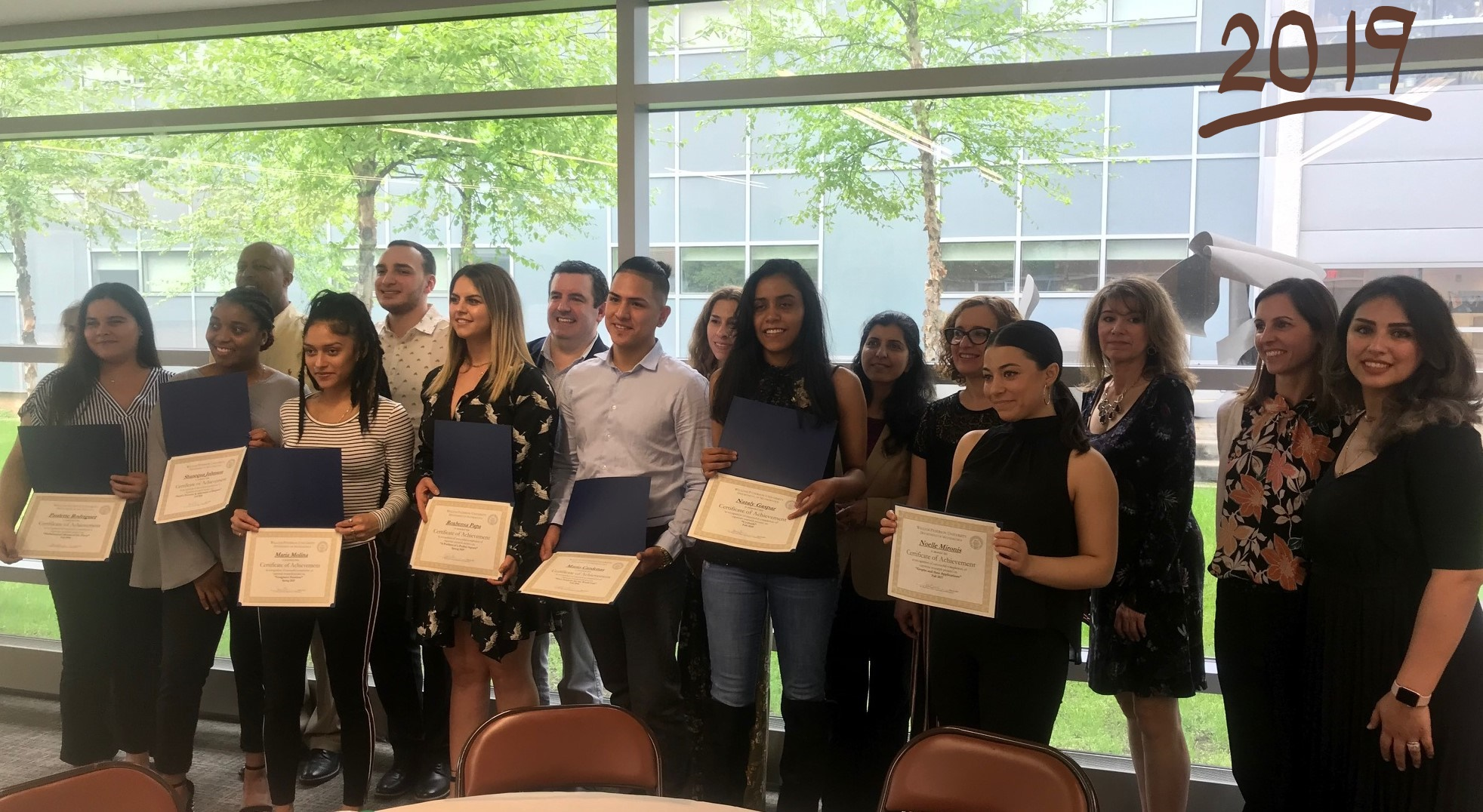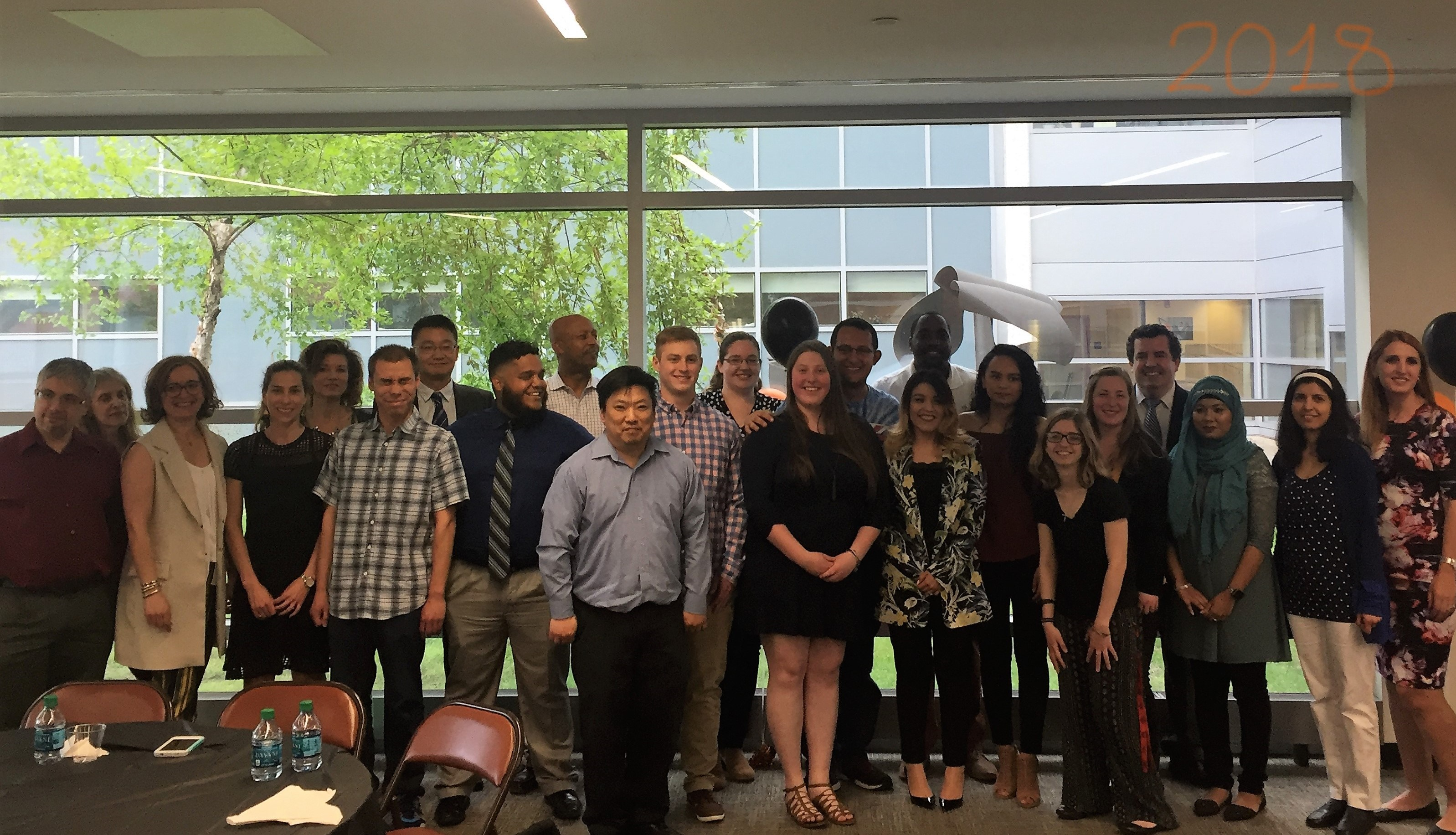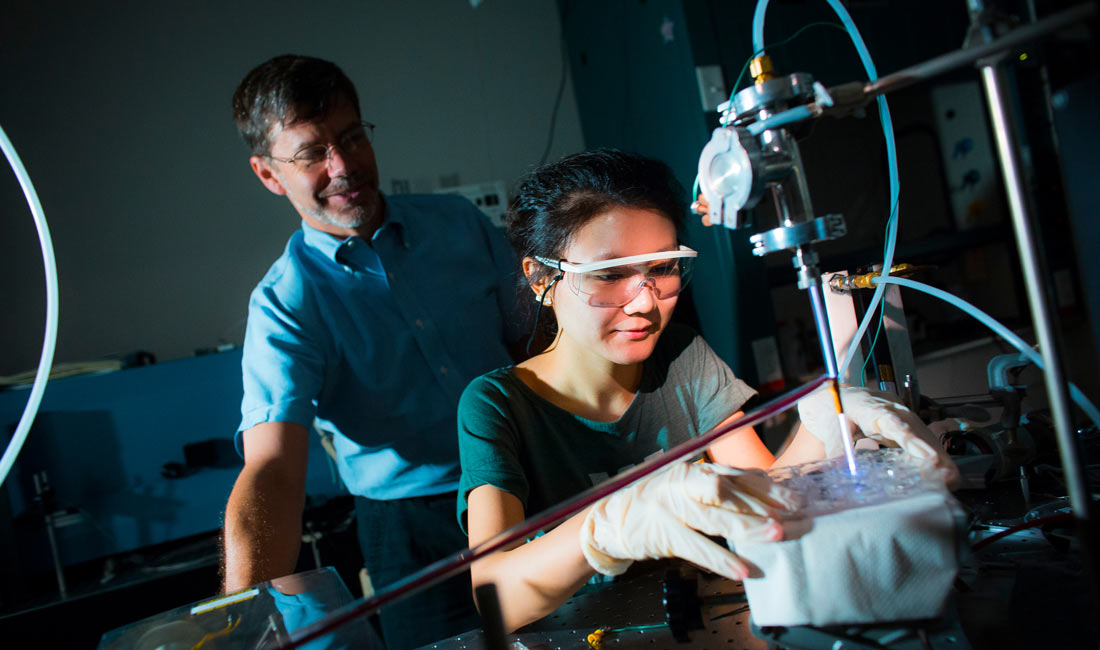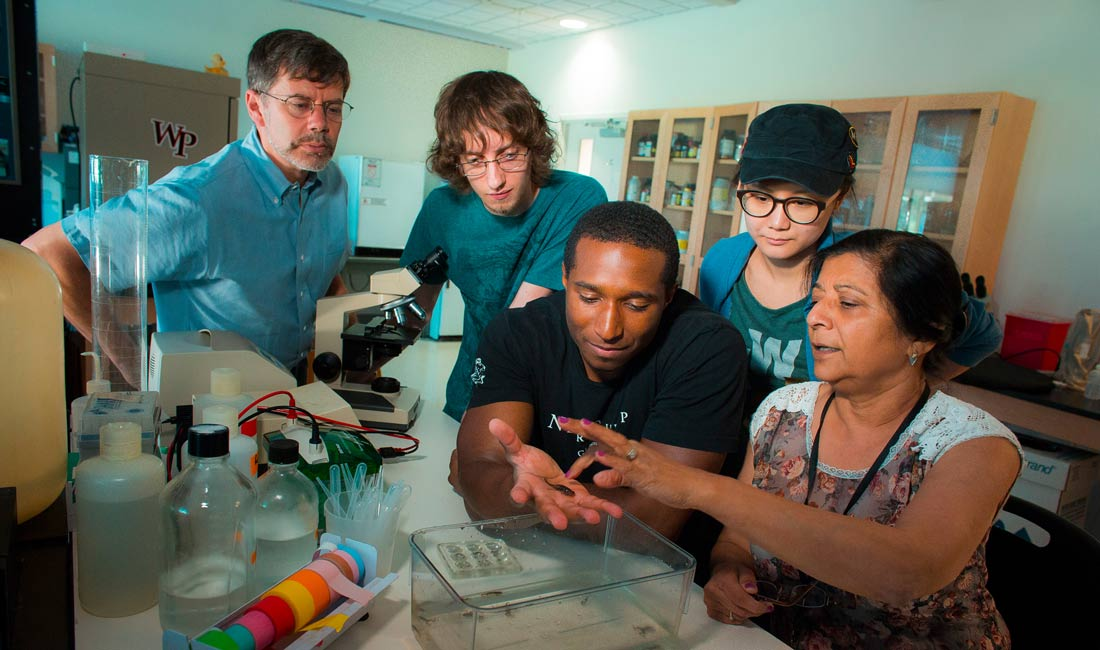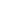The Department of Mathematical Sciences aspires to achieve an active and collaborative learning community where each member contributes to the learning of others. To develop such a learning community, the Department has an academically diversified faculty with specializations from many fields of mathematics and physics. The main goal of the Department is to allow students to function as scientists in the workplace and to prepare them for graduate school and teaching through its major and minor programs. The Department strives to develop competent, confident, enthusiastic students who have a broad foundation in the study of mathematical science and who can apply their developed skills and knowledge to a wide variety of theoretical and applied programs. For those students who are not science majors, it is our goal to provide exposure to the process of doing science and a broad knowledge of the field of physics along with applications of these sciences to everyday life.

• BA in Mathematics
• BS in Actuarial Science
• BS in Mathematics
• Minor in Mathematics
• Minor in Physics
• Minor in Statistics

• MS in Applied Mathematics

The bachelor of arts in mathematics will prepare a student well for a career in data analysis, actuary, or mathematics education. Any science, technology, or engineering career makes full use of a strong mathematics foundation. The bachelor of science in mathematics is well suited for engineering-related careers and for pursuing graduate school in mathematics. A minor in mathematics lays a strong foundation for those who wish to pursue higher education in the sciences or in business. A minor in statistics is advantageous to biostatistics, quality control, operations research, economic forecasting, marketing analysis and related professions.

Physics faculty conduct experimental and theoretical research, primarily in the fields of atomic, molecular, plasma, nuclear physics, and condensed matter. STEM students are strongly encouraged to participate in faculty-supervised research projects.  The research projects and the minor in physics will train you with excellent problem-solving skills that will in turn prepare you for success in graduate or professional schools and in STEM related careers.

Mathematics BS - Mission Statement:

Mission: The Department of Mathematics at William Paterson University aspires to achieve an active, collaborative learning community where each member contributes to the learning of others. To develop such a community for learning, the Department has an academically diversified faculty with specializations from many fields of Mathematics.

Mathematics - BS Student Learning Outcomes

1. Students will be able to organize and interpret data as well as various forms of graphs and charts.
2. Students will be able to undertake reasonably challenging mathematical tasks that require the interplay of modeling and problem-solving skills together with applications of mathematical theory and techniques.
3. Students will be able to undertake and formalize mathematical modeling designs of real-world problems, with the understanding of the important role of mathematics in all scientific disciplines as well as its increasing role in the social sciences.
4. Students will be able to make oral and written presentations using the language of mathematics in a precise and effective manner. Specifically, be able to research, organize, write and deliver a paper or a talk on a mathematical topic.
5. Students will be able to incorporate technology such as graphing calculators, Computer Algebra Systems (CAS), Internet, etc. appropriately.
6. Students will be able to have developed an understanding of the historical and contemporary role of mathematics in the context of other disciplines and society.
7. Students will be able to work effectively in groups and with each other.
8. Students will be able to be confident in the ability to be independent and lifelong learners of mathematics.
9. Students will be able to analyze polynomial, rational and transcendental functions or one or more variables.
10. Students will be able to demonstrate an understanding for applications of derivative and integral.
11. Students will be able to understand the fundamentals of vector space calculus.
12. Students will be able to understand the Theory of Linear Algebra.
13. Students will be able to organize and synthesize mathematical information into logical proofs.
14. Students will be able to communicate mathematical concepts, clearly and effectively in an organized manner.
15. Students will be able to communicate mathematical concepts, clearly and effectively in an organized manner.

Mathematics BS - Goals

1. Mathematical Thinking - Understand the logic of Mathematical Thinking
2. Mathematical Structures - Develop an understanding of Mathematics by studying underlying structures, relationships and operations
3.  Problem Solving - Develop a repertoire of problem-solving techniques
4. Application of Mathematics - Develop mathematical skills and applications
5.  Mathematical Rigor - Understand the difference between conjecture and rigorous mathematical proof
6. Mathematical expression - Use the language of mathematics to present precise descriptions and logical relationships

Mathematics BA - Mission Statement

The Department of Mathematics at William Paterson University aspires to achieve an active, collaborative learning community where each member contributes to the learning of others. To develop such a community for learning, the Department has an academically diversified faculty with specializations from many fields of Mathematics.

Mathematics BA - Goals

1. Understand the logic of mathematical thinking
2. Develop an understanding of Mathematics by studying underlying structures, relationships and operations
3. Develop a repertoire of problem-solving techniques
4. Develop mathematical skills and applications
5. Understand the difference between conjecture and rigorous mathematical proof
6. Use the language of mathematics to present precise descriptions and logical relationships

Mathematics BA - Student Learning Outcomes

1. Students will be able to organize and interpret data as well as various forms of graphs and charts.
2. Students will be able to undertake reasonably challenging mathematical tasks that require the interplay of modeling and problem-solving skills together with applications of mathematical theory and techniques.
3. Students will be able to undertake and formalize mathematical modeling designs of real-world problems, with the understanding of the important role of mathematics in all scientific disciplines as well as its increasing role in the social sciences.
4. Students will be able to make oral and written presentations using the language of mathematics in a precise and effective manner. Specifically, be able to research, organize, write and deliver a paper or a talk on a mathematical topic.
5. Students will be able to incorporate technology such as graphing calculators, Computer Algebra Systems (CAS), Internet, etc. appropriately.
6. Students will be able to have developed an understanding of the historical and contemporary role of mathematics in the context of other disciplines and society.
7. Students will be able to work effectively in groups and with each other.
8. Students will be able to be confident in the ability to be independent and lifelong learners of mathematics.
9. Students will be able to analyze polynomial, rational and transcendental functions or one or more variables.
10. Students will be able to demonstrate an understanding for applications of derivative and integral.
11. Students will be able to understand the fundamentals of vector space calculus.
12. Students will be able to understand the Theory of Linear Algebra.
13. Students will be able to organize and synthesize mathematical information into logical proofs.
14. Students will be able to communicate mathematical concepts, clearly and effectively in an organized manner.
15. Students will be able to communicate mathematical concepts, clearly and effectively in an organized manner.

Physics - Mission Statement

The Physics Department educates students to understand the concepts and phenomena of the physical world. The goal is to provide students in the STEM disciplines with a background of comprehensive knowledge and a set of skills that will allow them to function as scientists in the workplace or to be successful in graduate or professional schools.  For those students who are not science majors, it is our goal to provide exposure to the process of doing science and a broad knowledge of the field of physics along with applications of these sciences to everyday life.

Physics - Goals

The main goal of the Department is to serve STEM and liberal arts students, by the study of physics, to acquire critical- thinking skills and develop the understanding and problem-solving abilities which are increasingly needed in our technological society.  For students who wish to pursue pre-professional fields, such as pre-medicine, physics courses are essential for further advancement.

## Spotlight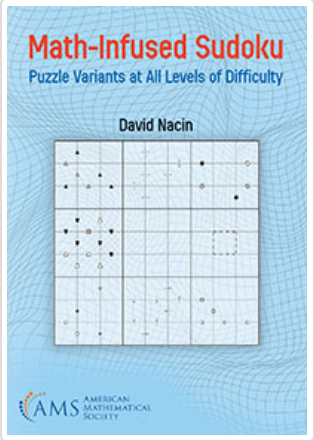The American Mathematical Society published Dr. David Nacin's book: Math-Infused Sudoku.